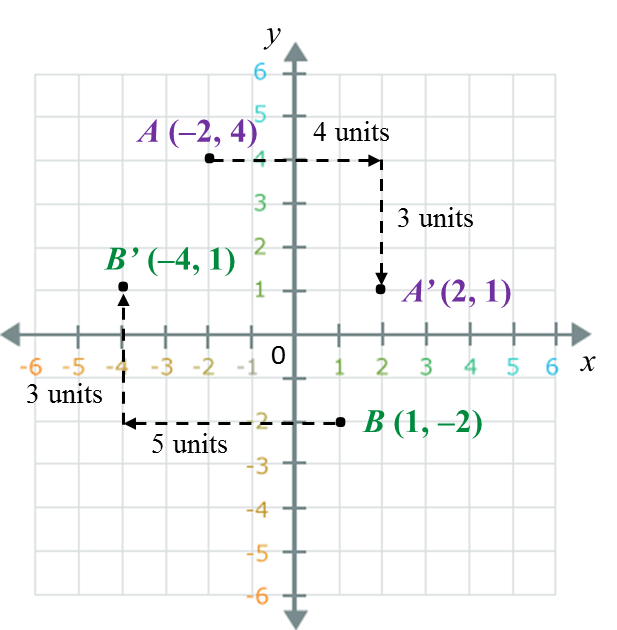# 11.1.1 Isometric Transformations

     
     
       
11.1 Isometric Transformations
        

    11.1.1 Transformation
 A transformation is a one-to-one correspondence or mapping between points of an object and its image on a plane.
 

    
 
           
       
11.1.2 Translation
       
1. A translation is a transformation which moves all the points on a plane through the same distance in the same direction.
  

   
       
2. Under a translation, the shape, size and orientation of object and its image are the same.
       

       
3. A translation in a Cartesian plane can be represented in the form         $\left(\begin{array}{l}a\\ b\end{array}\right),$          whereby, a represents the movement to the right or left which is parallel to the x-axis and b represents the movement upwards or downwards which is parallel to the y-axis.
       

       
Example 1:
       
Write the coordinates of the image of A (–2, 4) under a translation         $\left(\begin{array}{l}\text{}4\\ -3\end{array}\right)$          and B (1, –2) under a translation         $\left(\begin{array}{l}-5\\ \text{}3\end{array}\right)$         .
       

     Solution
:A’ = [–2 + 4, 4 + (–3)] = (2, 1)
       
B’ = [1 + (–5), –2 + 3] = (–4, 1)
       
       

     Example 2
:
       
Point moved to point K’ (3, 8) under a translation         $\left(\begin{array}{l}-4\\ \text{}3\end{array}\right).$        
       
What are the coordinates of point K?
       
       

     Solution
:
       
        $K\left(x,\text{}y\right)\to \left(\begin{array}{l}-4\\ \text{}3\end{array}\right)\to K\text{'}\left(3,\text{}8\right)$        
       
The coordinates of K = [3 – (– 4), 8 – 3]
       
= (7, 5)
 
          
Therefore the coordinates of K are (7, 5).
    
    
    
 
 

### 1 thought on “11.1.1 Isometric Transformations”

1.can you double check the example 2 ?? I think there was some mistake in the equation